# Test: Rectangles And Squares- 1

## 15 Questions MCQ Test Quantitative Aptitude for GMAT | Test: Rectangles And Squares- 1

Description
Attempt Test: Rectangles And Squares- 1 | 15 questions in 30 minutes | Mock test for GMAT preparation | Free important questions MCQ to study Quantitative Aptitude for GMAT for GMAT Exam | Download free PDF with solutions
QUESTION: 1

Solution:
QUESTION: 2

Solution:
QUESTION: 3

### Area of square ABCD is equal to the area of rectangle PQRS. If the length of rectangle PQRS is 21% more than its breadth, then what is the ratio of perimeter of ABCD to perimeter of PQRS?

Solution:
QUESTION: 4

What is the area of rectangle ABCD?

1. Length of each diagonal of rectangle ABCD = 13cm
2. Perimeter of rectangle ABCD = 34cm
Solution:
QUESTION: 5

A border of uniform width of ‘w’ inches is placed around a rectangular photograph. What is the area of the border, in square inches?

1. w = 2 inches
2. Perimeter of rectangular photograph = 20 inches
Solution:
QUESTION: 6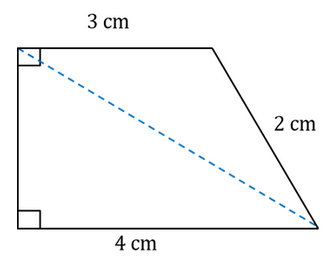In the given trapezium, what is the length of the diagonal shown by the dashed line?

Solution:

Step 1: Question statement and Inferences

Let’s label the trapezium as ABCD, where Angles A and B are right angles.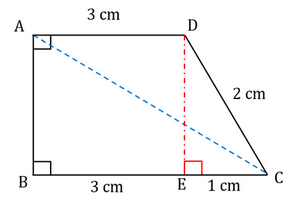To find the length of the diagonal, focus on the right triangle ABC. If we can find the length of side AB, we will be able to find the length of diagonal AC by applying the Pythagoras Theorem.

Step 2: Finding required values

Let us drop a perpendicular DE on the base BC.

So, BEDA is a rectangle. Therefore,

And, AB = DE

Now, BC = BE + EC

4 = 3 + EC

Therefore, EC = 1 cm

Let the length of side DE be h cm.

By applying Pythagoras Theorem in right triangle DEC, we get:

12 + h2 = 22

1 + h2 = 4

h2 = 3

h = √3 cm

Step 3: Calculating the final answer

AB = DE = √3 cm

Let the length of AC be d cm

Therefore, in right triangle ABC, by applying Pythagoras Theorem, we get:

(√3)2 + 42 = d2

3 + 16 = d2

19 = d2

d = √19

QUESTION: 7

The length of a rectangle is 9 cm more than its width, and the area of the rectangle is 486 cm2. What is the length of the diagonal (in cm) of the rectangle?

Solution: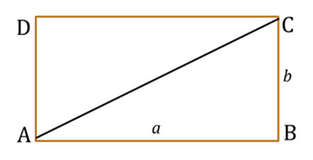To find – Length of Diagonal of the given rectangle.

Assumptions – Let the length of this rectangle be a cm and width be b cm.

Given

• Area of rectangle = 486 cm2
• a = b + 9

Solution

• We know the relationship between the diagonal and the length and width of the rectangle.
• Diagonal =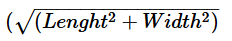• So we find the values of length and width, we will be able to calculate the diagonal.
• From the given information of the rectangle – area and the relationship between the length and the width, we should be able to find the values of length and width.

Area of rectangle – length x width

• a * b = 486
• Substituting a = b + 9 above we get:

• (b + 9) * (b) = 486
• b2 + 9b – 486 = 0
• (b+27)(b-18) = 0
• b = -27 and 18

Since b is a measure of length, it cannot be negative.  Thus b = 18.  This implies a = b + 9 = 27

Thus, diagonal can be calculated as follows:

Diagonal =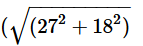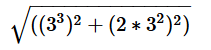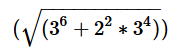=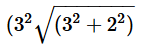=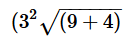=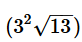QUESTION: 8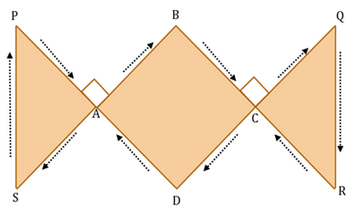A jogger runs along the track PABCQRCDASP.  How many times will he need to run around this track to cover 5 miles?  Note that ABCD is a square.

(1) ∠PSD = ∠QRD = 90° and PA = AB = CQ

(2) PS = QR = BD = 0.35 miles (rounded to nearest tenth)

Solution:

Steps 1 and 2 – Understand the question and draw inferences

To find – Number of rounds that the jogger will run around the path to cover 5 miles.

Given

• ∠PAB = ∠BCQ = 90°

Approach

Since we are given the total distance as 5 miles, to find the number of rounds taken by the jogger, we need to find the distance that the jogger will run in one round.  Thus, we need to find the perimeter of the path.

To find the perimeter of the path we need the measure of each individual side that make up the path.   Thus, we should look for information in the statements that help us arrive at the individual lengths of each side of the jogging path

Step 3 – Analyze Statement 1

Statement 1 indicates that ∠PSD = ∠QRD = 90° and PA = AB = CQ.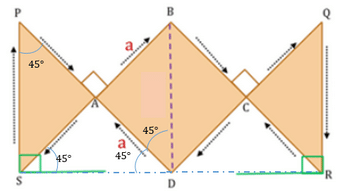So, we just need to find the value of a to calculate the perimeter of the path.

In triangle ABD, as ∠BAD = 900, using the Pythagoras theorem, we can write, 2a2 = 0.352

Thus, we can determine the value of a.  With this we have all the individual lengths along the jogging path.  Thus, we can determine the perimeter of the jogging path.

Thus, Statements (1) and (2) together yield a unique answer.

QUESTION: 9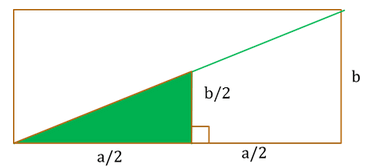If the shaded area represents a kids’ playground in a rectangular park, what is the perimeter of the rectangular park?

(1) At a rate of \$80 per meter, it costs \$1920 to fence the two smaller sides of the kids’ playground.

(2) The area of the kids’ playground is 64 m2.

Solution:

Steps 1 and 2 – Understand the question statement and draw inferences

To find – perimeter of rectangular park = 2 (a+b)

Given -The shaded area is as shown with the measurements in the figure.

• Base of triangular shaded area = a/2
• Height of triangular shaded area = b/2

Step 3 – Analyze Statement 1

At a rate of \$80 per meter, it costs \$1920 to fence the two smaller sides of the kids’ playground

Cost to fence = \$1920

Fencing rate = \$80/m

Therefore, length of the two smaller sides = 1920/ 80 = 24

So, we can write the equation: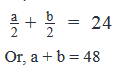• We know the sum of a and b
• Thus, we can find the value of perimeter of the rectangle as 2(a+b)

Statement 1 provides sufficient information to arrive at a unique answer.

Step 4 – Analyze Statement 2

The area of the kids’ playground is 64 m2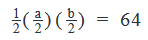or ab = 512

• This relationship gives us the product of a and b.
• It does not give us the individual values of a and b or the sum of a and b
• Thus, we cannot find the value of perimeter of the rectangle.

Statement 2 does not provide sufficient information to arrive at a unique answer.

Step 5 – Analyze Statement 1 and 2 together

Since statement 1 already provides sufficient information to arrive at a unique answer, we do not need to do step 5.

QUESTION: 10

What is the cost to fence a rectangular plot at the rate of \$20/meter?

(1) If length of the plot is increased by 10 meters, the area of the plot increases by 100 sq. m.

(2) If length of the plot is increased by 10% and width of the plot is decreased by 10%, the perimeter decreases by 5%.

Solution:

Steps 1 and 2 – Understand the question and draw inferences

To find – Cost to fence a rectangular plot at \$20/meter

Given

• Rectangular plot
• Rate of fencing -\$20/meter

Approach

• Fencing a rectangular plot implies going around its perimeter.
• So we need to find the perimeter of the rectangular plot.
• For this we need the value of the sum of the length and the width.

Step 3 – Analyze Statement (1)

Statement (1): If length of the plot is increased by 10 meters, the area of the plot increases by 100 sq. m.

Let’s assume the length and the width of the plot as ‘a’ and ‘b’ meters.

New Length = a + 10

New Area = (a+10) * b

Original Area = a * b

According to Statement (1):

(a + 10) * b = a * b + 100

Simplifying this further we get:

• ab + 10b = ab + 100
• 10b = 100
• b = 10

Even though we know the value of b, we cannot find the value of a since no other information is provided about the rectangular plot.

Thus, this statement does not provide any information about the sum of the length and the width and hence the information is not sufficient to arrive at a unique answer.

Step 4 – Analyze Statement (2)

Statement II: If length of the plot is increased by 10% and width of the plot is decreased by 10%, the perimeter decreases by 5%.

Let’s assume the length and width of the plot as ‘a’ and ‘b’ meters.

Original perimeter = 2(a + b)

New Length = a + 0.1a = 1.1a

New Width = b -0.1b = 0.9b

New perimeter = 2(1.1a + 0.9b)

According to Statement (2):

2(1.1a + 0.9b) = 0.95 (2(a + b))

• 1.1a + 0.9b = 0.95a + 0.95b
• 0.15a = 0.05b
• 3a = b

Even though we know the relationship between a and b, we cannot find the value of sum of a and b since no other information is provided.

Thus, this statement does not provide any information about the sum of length and width and hence the information is not sufficient to arrive at a unique answer.

Step 5 – Analyze both Statements Together

• Per Statement (1):  b = 10
• Per Statement (2):  3a = b

So combining these two statements, we can find individual values of a and b and hence we can find the sum of a and b.

Thus implies that we can find a unique answer to the question about determining the cost of fencing at the given rate.

Thus, Statements (1) and (2) together yield a unique answer.

Correct Answer Choice – Choice C

QUESTION: 11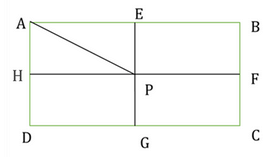ABCD is a rectangle whose longer side is AB. E, F, G and H are the mid-points of the sides AB, BC, CD and DA respectively. The line segment joining E and G intersects with the line segment joining F and H at point P. The perimeter of the rectangle EBFP is 28 units and the area of the triangle AEP is 24 square units. What is the length of each of the smaller sides, AD and BC, of the rectangle ABCD?

Solution:

Step 1: Question statement and Inferences

Since EG is joining the midpoints of the lengths of the rectangle, it is parallel to AD and BC. (i.e., EG is perpendicular to AB and CD).

Similarly, FH is parallel to AB and CD. (FH is perpendicular to AD and BC).

Both EG and FH bisect each other at the point P.

Let the length of AE and EP be x and y respectively.

Area = 24

½ * x * y= 24

xy = 48 ------equation (1)

Note that AE = EB = HP = PF = DG = GC = x

Similarly, AH = HD = EP = PG = BF = FC = y

Perimeter of EBFP = 28

2*(x + y) = 28

(x + y) = 14 ------Equation (2)

Step 2:Finding required values

Upon substituting x = 48/y from equation (2) in equation (1),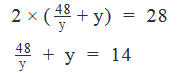Multiplying with y on both sides of the equation, we have

y2 – 14y + 48 = 0

y2 – 8y – 6y + 48 = 0

(y – 8)(y – 6) = 0

y = 8 or y = 6

Step 3: Calculating the final answer

Substituting the value of y in x = 48/y, to obtain the value of x.

If y = 8, then x = 6 and if y = 6, then x = 8.

The longer dimension is 8 units while the smaller dimension is 6 units.

Therefore BF = 6

The smaller of the sides of the rectangle = BC = 2 * 6 = 12 units

QUESTION: 12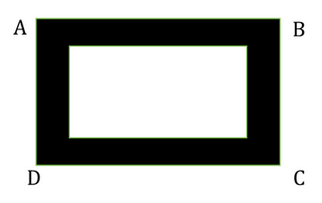A rectangular photograph shown by the white region in the figure is enclosed by a frame of uniform width. The shaded region in the figure represents the frame. What is the ratio of AB to BC?

(1) The ratio of the longer dimension to the smaller dimension of the photograph is 3:2.

(2) If a frame of twice the width was used then the ratio of AB to BC would have been 5:4.

Solution:

Steps 1 & 2: Understand Question and Draw Inferences

Notice that the frame has the same width throughout the border.

Let us say that the longer dimension of the photograph is L, the smaller dimension of the photograph is B and the width of the frame is x.

Then, AB = L + 2x

And, BC = B + 2x

We need to find the ratio of AB:BC. To do so, we ought to know the values of L, B, and x.

Step 3: Analyze Statement 1

The ratio of the longer dimension to the smaller dimension of the photograph is 3:2

Let’s say that for the white area the length of the longer dimension and the length of the shorter dimension are 3z and 2z where z is a positive number.

Thus,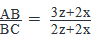With different values of z and x, we shall obtain different values of the required ratio.

For example, if z =1, x = 1, then AB:BC = 5:4. Whereas, if z = 1, x = 2, then AB:BC = 7:6.

INSUFFICIENT.

Step 4: Analyze Statement 2

If a frame of twice the width was used then the ratio of AB to BC would have been 5:4

In this case, AB = L + 2(2x) = L + 4x

And, BC = B + 2(2x) = B + 4x

We are given that AB:BC = 5 : 4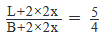We need to find the ratio of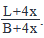Without the values of L, B, and x, it is impossible to determine its value.

INSUFFICIENT.

Step 5: Analyze Both Statements Together (if needed)

Combining statements (1) and (2),

We know that L:B = 3:2, but we do not know the values of L and B.

Let’s assume the values of L and B to be 3z and 2z and the original width is x.

Then,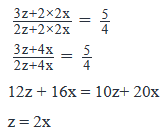Required ratio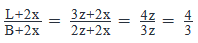The ratio has been determined.

SUFFICIENT.

QUESTION: 13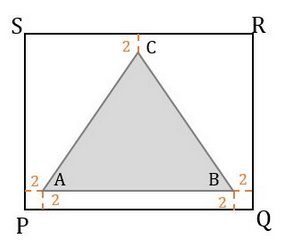A triangle ABC is placed inside a rectangle PQRS such that the minimum distance of any vertex of the triangle from the rectangle is 2 inches. What is the area of the rectangle PQRS?

(1) Triangle ABC is an equilateral triangle

(2) The area of Triangle ABC is 4√3 square inches and the length of the side AB of the triangle is 50% of the length of side PQ of the rectangle.

Solution:

Steps 1 and 2 – Understand the question statement and draw inferences

To find – Area of rectangle PQRS given the conditions about triangle ABC.

Given – Minimum distance from any vertex of the triangle to the frame = 2”.

The Area of the Rectangle = PQ*PS . . . (1)

So, in order to find the area, we need to know the values of PQ and PS.

Now, from the given diagram, it’s clear that PQ = 2 + AB  +2

That is, PQ = AB + 4 . . . (2)

Similarly, let’s try to express PS in terms of a dimension of the triangle ABC. (Note: We are expressing the dimensions of the rectangle in terms of the dimensions of the triangle because both St. 1 and 2 give us information regarding the triangle only)

Let’s drop a perpendicular CD from C on the side AB.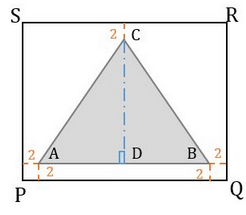By doing so, we get: PS = 2 + CD + 2

That is, PS = CD + 4 . . . (3)

Substituting (2) and (3) in (1), we get:

Area of rectangle = (AB+4)(CD+4) . . . (4)

Thus, in order to find the area of rectangle PQRS, we need to find the values of AB and CD.

With this understanding, let’s analyze the given statements.

Step 3 – Analyse Statement (1)

Per statement (1), Triangle ABC is an equilateral triangle.

This means, AB = BC = AC

And, CD = √3/2 * AB

However, since this statement doesn’t provide us the exact value of AB, we will not be able to find the area of rectangle PQRS.

Therefore, statement 1 does not provide sufficient information to arrive at a unique answer.

Step 4 – Analyse Statement (2)

Statement 2 provides two pieces of information

• Area of Triangle ABC = 4√3  in2.
• AB = 50% of PQ.

Area of triangle ABC = ½ * AB * CD = 4√3

• AB * CD = 8√3 ….(5)

Also,

• AB = 0.5 * PQ
• 2AB = AB + 4
• AB = 4 . . . (6)

Substituting (6) in (5), we get:

4CD = 8√3

So, CD = 2√3 .  .  .(7)

Substituting (6) and (7) in (4), we can find the area of rectangle PQRS.

Thus, St. 2 is sufficient to determine the area of the rectangle.

Step 5 – Analyze both Statements together

This step is not required since statement 2 helps us arrive at a unique answer.

QUESTION: 14

The floor of a company's storage room has an area, of 20,000 square feet. If the floor is in the shape of a square, approximately how many feet long is each side?

Solution:

Factorize 20000 to its prime we get

20000=2∗2∗2∗2∗2∗5∗5∗5∗520000=2∗2∗2∗2∗2∗5∗5∗5∗5
or, 20000=24∗2∗5420000=24∗2∗54
Taking square root of this we get 22∗52∗222∗52∗2
which is equal to 100√2

QUESTION: 15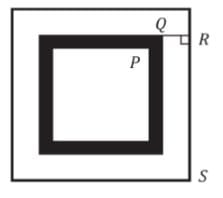squares are placed one inside another, leaving a strip of uniform width around each square. If

PQ = 2√2 units, QR = 2 units and RS = 10 units, what is the area of the shaded region?

Solution:

Width of strip is uniform around each square. PQ = 2√2 units, QR = 2 units and RS = 10 units.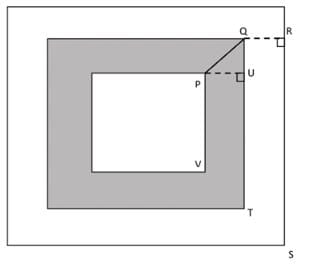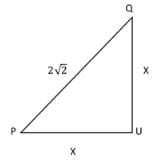irst of all we will draw a perpendicular PU from vertex P on side QT.
So in Triangle PUQ:-
Let PU = QU = x units. (width of the shaded region)
By applying Pythagoras theorem,
(PQ)2 = (PU)2 + (QU)2
(2√2)2 = x2 + x2
2x2 = 8
x2 = 4
x = 2 units.
As, RS =10 units.
So, QT = RS – QR = 10 – 2 = 8 units.
And, PV = QT – 2(PU) = 8-2(2) =4 units.
Area of Shaded region = (Area of square with side QT) – (Area of square with side PV) = (8 × 8) – (4 × 4)= 48 sq units.Use Code STAYHOME200 and get INR 200 additional OFF Use Coupon Code